Download Presentation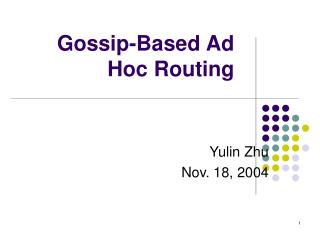Gossip-Based Ad Hoc Routing

# Gossip-Based Ad Hoc Routing - PowerPoint PPT Presentation

Gossip-Based Ad Hoc Routing. Yulin Zhu Nov. 18, 2004. Contents. Introduction Pure Gossip Optimization of Gossip Incorporate Gossip in AODV Parametric Probabilistic Sensor Network Routing Summary. Ad Hoc Network.I am the owner, or an agent authorized to act on behalf of the owner, of the copyrighted work described.
Download Presentation## Gossip-Based Ad Hoc Routing

An Image/Link below is provided (as is) to download presentation

Download Policy: Content on the Website is provided to you AS IS for your information and personal use and may not be sold / licensed / shared on other websites without getting consent from its author.While downloading, if for some reason you are not able to download a presentation, the publisher may have deleted the file from their server.

- - - - - - - - - - - - - - - - - - - - - - - - - - E N D - - - - - - - - - - - - - - - - - - - - - - - - - -
Presentation Transcript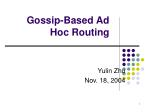### Gossip-Based Ad Hoc Routing

Yulin Zhu

Nov. 18, 2004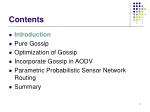Contents
• Introduction
• Pure Gossip
• Optimization of Gossip
• Incorporate Gossip in AODV
• Parametric Probabilistic Sensor Network Routing
• Summary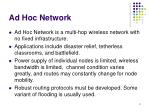• Ad Hoc Network is a multi-hop wireless network with no fixed infrastructure.
• Applications include disaster relief, tetherless classrooms, and battlefield.
• Power supply of individual nodes is limited, wireless bandwidth is limited, channel condition varies greatly, and routes may constantly change for node mobility.
• Robust routing protocols must be developed. Some variant of flooding is usually used.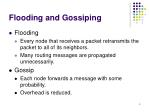Flooding and Gossiping
• Flooding
• Every node that receives a packet retransmits the packet to all of its neighbors.
• Many routing messages are propagated unnecessarily.
• Gossip
• Each node forwards a message with some probability.
• Overhead is reduced.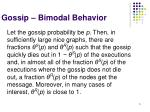Gossip – Bimodal Behavior

Let the gossip probability be p. Then, in sufficiently large nice graphs, there are fractions θS(p) and θR(p) such that the gossip quickly diesout in 1 − θS(p) of the executions and, in almost all of the fraction θS(p) of the executions where the gossip does not die out, afraction θR(p) of the nodes get the message. Moreover, in manycases of interest, θR(p) is close to 1.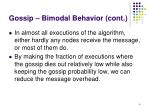Gossip – Bimodal Behavior (cont.)
• In almost all executions of the algorithm, either hardly any nodes receive the message, or most of them do.
• By making the fraction of executions where the gossip dies out relatively low while also keeping the gossip probability low, we can reduce the message overhead.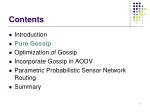Contents
• Introduction
• Pure Gossip
• Optimization of Gossip
• Incorporate Gossip in AODV
• Parametric Probabilistic Sensor Network Routing
• Summary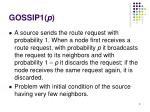GOSSIP1(p)
• A source sends the route request with probability 1. When a node first receives a route request, with probability p it broadcasts the request to its neighbors and with probability 1 – p it discards the request; if the node receives the same request again, it is discarded.
• Problem with initial condition of the source having very few neighbors.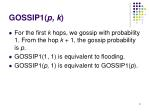GOSSIP1(p, k)
• For the first k hops, we gossip with probability 1. From the hop k + 1, the gossip probability is p.
• GOSSIP1(1, 1) is equivalent to flooding.
• GOSSIP1(p, 1) is equivalent to GOSSIP1(p).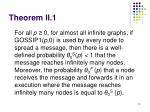Theorem II.1

For all p ≥ 0, for almost all infinite graphs, if GOSSIP1(p,0) is used by every node to spread a message, then there is a well-defined probability θ0S(p) < 1 that the message reaches infinitely many nodes. Moreover, the probability θ0F (p) that a node receives the message and forwards it in an execution where the message reaches infinitely many nodes is equal to θ0S (p).Cont.
• θ0S(p) = θ0F (p) =defθ0(p)
• In an execution where the message does not die out, the probability that a random node receives the message is θ0(p)/p.For GOSSIP1(p, k)
• θkS(p) – Probability that a message reaches infinitely many nodes.
• θ1S(p) = θ0(p) / p
• Given that a message doesn’t die out, the probability that a node receives and forwards the message is still θ0(p).Bimodal Behavior
• Either hardly any nodes get the message, or a fraction θ0(p) / p receive the message.
• In cases of interest, θ0(p) is quite close to p. Thus, in almost all executions of the algorithm in sufficiently large graphs, either hardly any nodes receive the message, or most do.Experiment – Probability varies

Gossiping on a random network of average degree 8.

The higher the probability, the higher the fraction of nodes receive the message.Experiment - Probability varies

Gossiping on a random network of average degree 8.

The higher the probability, the higher the fraction of nodes receive the message.Experiment – Degree of network
• In a 20 × 50 regular network of degree 6, gossiping with probability .65 ensure that almost all nodes get the message in almost all executions.
• for a 20 × 50 regular network of degree 3, we need to gossip with probability .86 to ensure that almost all nodes get the message in all executions.

Conclusion: the higher the degree, the better the gossiping effect.Experiment - θkS(p) with p

A critical probability p can be chosen so that almost all the executions don’t die out.Experiment - θkS(p) and θkR(p) with k
• θkR(p) = θ0(p) / p – doesn’t change with a fixed p.
• θ1S(p) = θ0S(p) / p.
• θ1S(.65) = .95, θ2S(.65) = .98, θ5S(.65) = 1.
• θ1S(.6) = .53, θ4S(.6) = .67, θ10S(.6) = .73.

Conclusion:

- As k goes from 0 to 1, there is a significant increase of θkS(p).

- As k increases beyond 1, there is increase in θkS(p), but it is not significant.GOSSIP1(p, k) - Conclusion
• With p sufficiently high, we can guarantee that almost all nodes will receive the message in almost all executions.
• Practically, we can guarantee that the destination node receives the message, while saving a fraction of 1 – p of messages.
• In cases of interest, the probability is about .65 - .75. All nodes get the message using 25-35% fewer messages than flooding.Contents
• Introduction
• Pure Gossip
• Optimization of Gossip
• Incorporate Gossip in AODV
• Parametric Probabilistic Sensor Network Routing
• SummaryA two-threshold scheme

Why?

In a random network, a node may have very few neighbors, thus the probability that none of the node’s neighbors will propagate the gossip is high. We hope that nodes with lower degree can gossip with higher probability.GOSSIP2(p1, k, p2, n)
• p1 – typical gossip probability.
• k – number of hops with which we gossip with probability 1.
• n – number of neighbors of a node.
• p2 – probability for which p2 > p1. Neighbors of a node with fewer than n neighbors gossip with probability p2 instead of p1.Comparison of GOSSIP2 with GOSSIP1

GOSSIP2 vs. GOSSIP1 on a random network of average degree 8

GOSSIP2(0.6,4,1,6) has better performance than GOSSIP1(0.75,4), while using 4% fewer messages than GOSSIP1(0.75,4).Prevent premature gossip death

The idea behind:

If a node has n neighbors and the gossip probability is p, for each message, the node should get roughly pn copies from its neighbors. If the node gets significantly fewer than pn copies within a reasonable time interval, then this is a clue that the message is dying out.GOSSIP3(p, k, m)

Same as GOSSIP1(p, k) except for the following modification:

If a node originally did not broadcast a received message, but then did not get the message from at least m other nodes within some timeout period, then the node will broadcast the message immediately after the timeout period.

Usually m = 1.Comparison of GOSSIP3 with GOSSIP1

GOSSIP3 vs. GOSSIP1 on a random network of average degree 8

GOSSIP3(0.65,4,1) has better performance than GOSSIP1(0.75,4), while using 8% fewer messages than GOSSIP1(0.75,4).Contents
• Introduction
• Pure Gossip
• Optimization of Gossip
• Incorporate Gossip in AODV
• Parametric Probabilistic Sensor Network Routing
• SummaryOverview of AODV

Using AODV, the first time a node u requests a route to node v, it uses an expanding-ring search to find the route. That is, it first tries to find the route in a zone of small radius, by flooding. It then tries to find the route in zones of larger and larger radius. If all these attempts fail, it resorts to flooding the message throughout the whole network. The exact choice of zone radii to try is a parameter of AODV. Typically, not too many radii are considered before resorting to flooding throughout the network.

AODV also maintains a routing table where it stores the route after it has been found. Every time a packet is sent, the route in the routing table is tried first.AODV + G (AODV using GOSSIP3)

In AODV, if the expanding-ring search with a smaller radius fails, rather than flooding to the whole network, we use GOSSIP3.

In the experiment, GOSSIP3(.65, 1, 1) is used.Comparison of AODV+G and AODV

Pause time – the time a node pauses after reaching a randomly set destination. Then the node moves again.Comparison of AODV+G and AODV (cont.)

Normalized routing load – the number of routing packets transmitted per data packet delivered at the destination.Comparison of AODV+G and AODV (cont.)

Route length ratio – the ratio of the actual shortest route length to the shortest route length found.Contents
• Introduction
• Pure Gossip
• Optimization of Gossip
• Incorporate Gossip in AODV
• Parametric Probabilistic Sensor Network Routing
• SummaryParametric Probabilistic Sensor Network Routing

Christopher L. Barrett, Stephan J. Eidenbenz, Lukas Kroc,

Madhav Marathe, James P. Smith (WSNA’03)

DESTINATION ATTRACTOR - the retransmission probability at a node depends on the distance counted in hops from the source node to the destination node and the hop-distance from the node currently holding a packet to the destination.

DIRECTED TRANSMISSION - the probability depends on the same two distance factors and additionally on the number of hops that the packet has already traveled.Notation
• S – source sensor.
• D – destination sensor.
• i – number of time steps.
• Ri – a sensor holding a packet at time step i.
• Ri-1 – the sensor that sent the packet to Ri.
• d(Ri, Rj) – hop distance between Ri and Rj.
• PRi – retransmission probability of Ri.

At time step i, all sensors who received a packet at the previous time step i-1 decide whether to retransmit the packet, and, if affirmative, do so.DESTINATION ATTRACTOR

Idea behind:

If the packet is getting closer to the destination, then its retransmission probability is increased; if it is getting further away from the destination, then the retransmission probability is reduced.DIRECTED TRANSMISSION

Idea behind:

The nodes that lie on a shortest path from the source to the destination should forward packets with a very high probability and the farther away a node is from the shortest path, the smaller its retransmission probability should be.DIRECTED TRANSMISSION (cont.)

Let Ri' denote any node that holds a packet at time i. The node minimizing minRi'd(Ri', D) is the node closest to the destination. Thus:Experiment
• Noise is used to model sensor mobility, node failure and other inaccuracy.
• Protocols tested in the experiment are as follows:Experiment (cont.)

Experiment Setup:

- 5000 sensors are spread uniformly at random in a square field, where all sensors have equal range of transmission, inducing a graph with average node degree of approximately 6.7.

- A TDMA model is assumed for the medium access control (MAC) sublayer.Experiment Result

Load - number of times any sensor transmits a packet.

Lag - number of time steps it takes to deliver the packet to the destination.

Fraction delivered - fraction of runs where the destination receives the data.

The Lag is divided by length of the shortest path from source to destination for each run to make the runs comparable.Experiment Result (cont.)

The parameter of Directed Transmission and Destination Attractor is k.

The parameter of Gossiping is p.Conclusion
• DESTINATION ATTRACTOR and DIRECTED TRANSMISSION can guarantee any quality of service level (measured as fraction delivered) even in the presence of highly noised network information.
• Multi-path methods outperform single-path methods in the presence of noise.
• Making the retransmission probability depend on network information parameters is a promising step towards more robust routing protocols in sensor networks.Contents
• Introduction
• Pure Gossip
• Optimization of Gossip
• Incorporate Gossip in AODV
• Parametric Probabilistic Sensor Network Routing
• SummarySummary
• Pure Gossip (GOSSIP1).
• Optimization of Gossip (GOSSIP2 and GOSSIP3).
• Integrate Gossip with AODV.
• Parametric Probabilistic Sensor Network Routing.References
• Zygmunt Haas, Joseph Y. Halpern, Li Li. Gossip-Based Ad Hoc Routing. Proceedings of INFOCOM 2002, 2002.
• Christopher L. Barrett, Stephan J. Eidenbenz, Lukas Kroc, Madhav Marathe, James P. Smith. Parametric Probabilistic Sensor Network Routing. WSNA'03.
• P.Th.Eugster, R.Guerraoui, S.B.Handurukande, A.M.Kermarrec, P.Kouznetsov. Lightweight Probabilistic Broadcast. DSN'01.
• L. Rodrigues, S. Handurukande, J. Pereira, R. Guerraoui, A.-M. Kermarrec. Adaptive Gossip-Based Broadcast. DSN'03.
• Werner Vogels, Robbert van Renesse, Ken Birman. The Power of Epidemics: Robust Communication for Large-Scale Distributed Systems. ACN SIGCOMM, Volume 33, Number 1: January 2003.